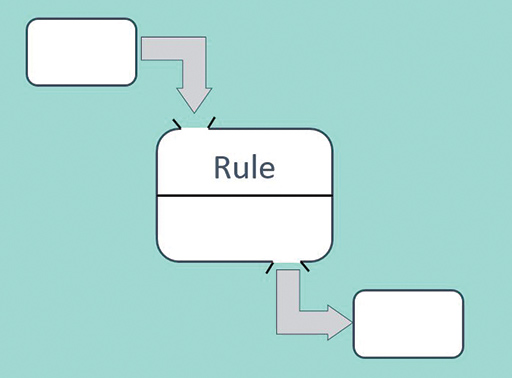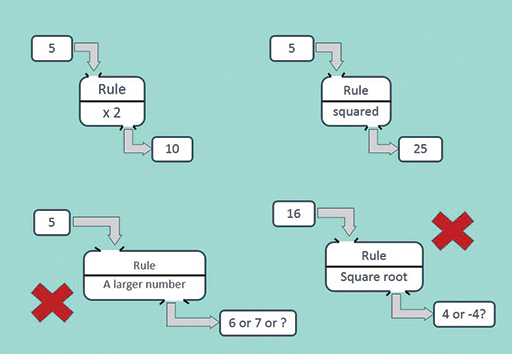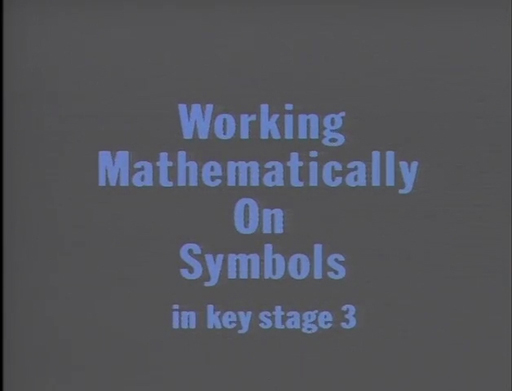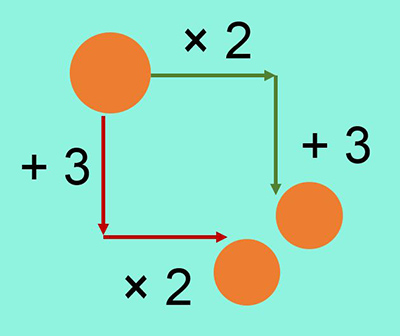Teaching mathematics

Start this free course now. Just create an account and sign in. Enrol and complete the course for a free statement of participation or digital badge if available.

Free course

# 1 Function machines

The function machine is a way of thinking about three aspects that make up a function: the inputs, the outputs and the rule that determines their connection (Figure 2). The representation as a machine connects them in one diagram (just like seeing a toaster makes you think of bread and toast).

You have already seen examples of such thinking in the ‘Think of a Number’ problems. The input is the number you think of, you calculate given a rule and you tell someone the answer – the output.Figure 2 Function machine

The rules for functions can be as simple as ‘double’ or ‘stay the same’ and as complicated as you like. (It does not even have to be expressed in symbols.) The rule does have to be ‘well defined’, meaning that for each input number there is only one possible answer.

This condition means that two of the examples in Figure 3 are not functions:Figure 3 Examples and non-examples of functions

As you saw with Think of a Number problems, the function machine representation is powerful because learners can work with it at the level of taking a specific unknown quantity – the input – and calculating with it to get an output. In this sense, the function is a process that they can carry out.

The machine representation also lends itself to more sophisticated tasks requiring learners to go beyond simply finding outputs. Teachers can introduce the idea of the input as a quantity that can take any value. They can set tasks where the process of writing and comparing the rules and outputs is more important than what numbers they are. The two activities you will carry out in this section illustrate this kind of task.

The goal of working in this way is laying the foundations for learners to treat quantities as variables and to use functions fluently in later mathematics.

For example:

• the squaring function is used in finding the area of a circle
• the cubing function is used in finding volume
• the sine function is used in trigonometry (and, of course, the cosine and tangent functions).

The ‘machine’ analogy suits these examples since it corresponds to learners’ experiences of pressing the calculator key for that function (Figure 4). Before calculator technology, all of these functions were either calculated or looked up in special booklets of tables.Figure 4 Functions on your calculator

## Activity 1 Getting used to long expressions

Timing: Allow 10 minutes

On a piece of paper, write an expression that summarises the following sequence of functions.

Think of a number, then:

• multiply by 2
• subtract 5
• divide by 3
• multiply by 6.

Then watch the video below from the OU archives. It introduces a teacher, and shows him building up the sequence with 14-year-old learners on a board. Ask yourself:

What do you think the teacher’s learning objectives are?

How do learners react to calculating on an unknown quantity?

What does the teacher do to draw attention to conventional symbol use?

Download this video clip.Video player: Video 1 Working mathematically with symbols at Key Stage 3Skip transcript: Video 1 Working mathematically with symbols at Key Stage 3

#### Transcript: Video 1 Working mathematically with symbols at Key Stage 3

DAVE HEWITT:
When I first started teaching, I entered into a school where the diet was mainly textbooks or work cards. And I felt that the way the pupils were being asked to work was getting them to do rather repetitive tasks and to always seek an authority somewhere, whether it is me or whether it is the answers at the back of the book.
I was very unhappy with that. And I worked quite hard at working with them in a different way where I tried to find out what they could do themselves. They have minds they bring with them, all sorts of experiences and abilities. And I spent quite a long time, many years, in classrooms finding out what they can do if left, in a sense, to their own devices.
And I found that the pupils realised that they can be quite creative in mathematics and that they are functioning mathematicians at their level. And I saw many pupils grow as a consequence of that. And quite often, I would arrive in a classroom. And if I happened to be a bit late, then quite often, they were working already and they were quite autonomous in that sense.
I then also began to ask the question, well, what is my role? And I felt that I wanted to see whether I could possibly accelerate this learning so that they might be able to learn faster just as comfortably. And as a consequence, I think I've begun working in ways where I'm quite controlling what's happening in the classroom. I'm very much controlling it. And in many ways, the activity relies on me as a controlling body in that room.
I hope, and I feel that I succeed, in still making them realise that they are still in control. So I'm not telling them what to do, but I am trying to affect their attention and perhaps direct their attention to different things, so that they can notice things, perhaps a little bit quicker than if their attention wasn't drawn to certain things.
These lessons are not every lesson. I won't control it in that way every lesson. It's quite often just a starter that may last for one or two or three lessons at the most, I would have thought. What am I going to do to this one? Oh, yes, I'm going to add 3, times by 2, take away 5, divide by 3, add 72.
Got a problem with this? Do you want me to write down what I'm doing?
STUDENTS:
Yes.
DAVE HEWITT:
So-- let me see. I'm thinking of a number. I add 3. Then I'm going to multiply by 2. Then I'm going to take away 5. Then I'm going to divide by 3. Then I'm going to add 72. And then I'm going to multiply by 6. And I get 100. So you're going to-- Jemma.
STUDENT 1:
100 divided by 6.
DAVE HEWITT:
100--
STUDENT 1:
Divide by 6.
DAVE HEWITT:
And you're dividing by 6 because?
STUDENT 1:
Because it was times 6. And so you take that and it's divide by 6.
DAVE HEWITT:
So that's done.
STUDENT 1:
Yeah. Then take 72.
DAVE HEWITT:
OK, and after that-- I've done that.
STUDENT 1:
Times 3.
DAVE HEWITT:
Because I--
STUDENT 1:
Divide 3.
DAVE HEWITT:
So you're going to--
STUDENT 1:
It's times 3.
DAVE HEWITT:
Times by-- all right. We're left with this.
STUDENT 1:
Divide it by 2. Or no-- add 5.
STUDENT 2:
Add 5, divide it by 2.
DAVE HEWITT:
STUDENT 1:
5.
DAVE HEWITT:
Right. So that's that done.
STUDENT 1:
Divide by 2.
DAVE HEWITT:
Divide by 2.
STUDENT 1:
Take 3.
DAVE HEWITT:
And take 3. And I end up with-- when I first started off saying I'm thinking a number, what did I write down?
STUDENT 1:
x.
DAVE HEWITT:
So I end up with whatever that number is, and we could work it out and find out what it is. All right.
End transcript: Video 1 Working mathematically with symbols at Key Stage 3
Video 1 Working mathematically with symbols at Key Stage 3
Interactive feature not available in single page view (see it in standard view).

### Discussion

The emphasis of the video activity is on gaining familiarity with symbols to express the whole sequence without any intermediate results being calculated. Note how the teacher builds up the expression first by ‘doing’ each function, and then by ‘undoing’ it. ‘Doing and undoing’ is an important idea in algebra. It provides an organising structure to relate processes that appear different but are the reverse of each other, such as × and ÷. This allows us to reduce the complexity of calculations.

The next activity is a well-tested teaching approach devised by Don Steward, called ‘both ways’. Using two functions (× 2 and + 3) increases the level of complexity, and going both ways allows learners to make predictions about the results. This task is designed to shift attention from calculating outputs towards comparing methods of calculation. The activity is written in the way you would use it with a class of learners, although you would need to offer support for some of the calculations (for example, a negative number line).

## Activity 2 Both ways

Timing: Allow 10 minutesFigure 5 Both ways

You are going to choose an input number and follow the instructions on the arrows in Figure 5 – both ways. Do you think your answers will be the same?

• Try starting with these numbers: 5, 8, 3.1, –4, 100, 1000
• a million (you could write m for short)
• a billion (b for short)
• any number (n for short)
• Compare the two answers you get. Can you explain what is happening?

### Discussion

You get different answers by going different ways:

13 (green way) and 16 (red way), 19 and 22, 9.2 and 12.2, –5 and –2, 203 and 206, 2m+3 and 2m+6, etc.

The difference between the answers is always 3, for any input number.

Using m for a million, etc. is a popular way of introducing letters for numbers in function machines. It matches the way we speak about such numbers in words: 2 million and 3. It is mathematically correct in that m, b, t all stand for numbers – one million, one billion, etc. – rather than objects such as mangos or bananas that cannot be calculated with.

Although it is a good introduction, it is not an approach to stay with. (For example, you do not want learners to write 2 million one hundred and three as 2m1h+ 3 or think that m always stands for a million.) This is why the sequence of examples ends with using n for ‘any number’ (the sound rhyme helps learners accept this meaning).

You could explain this result with diagrams using a rectangle for n and dots for each 1. The important step is that, following the red arrows, both the number and the 3 dots get multiplied by 2.

This activity shows the need to use brackets when you combine addition and multiplication in a single expression. If you are confident with algebra, you might have explained it to yourself by writing the expression for each way and expanding the brackets. However, learners in middle school are unlikely to be convinced by manipulating symbols because they do not yet feel concrete to them.

green arrows 2n+3

red arrows 2(n+3) = 2 × n + 2 × 3 = 2n + 6

2n and 2n are the same number, but 6 is more than 3 so the difference is 3.

You can find resources related to this activity on the Median blog at https://donsteward.blogspot.com/ [Tip: hold Ctrl and click a link to open it in a new tab. (Hide tip)] indexed under ‘Expressions Both Ways’.

TM_1